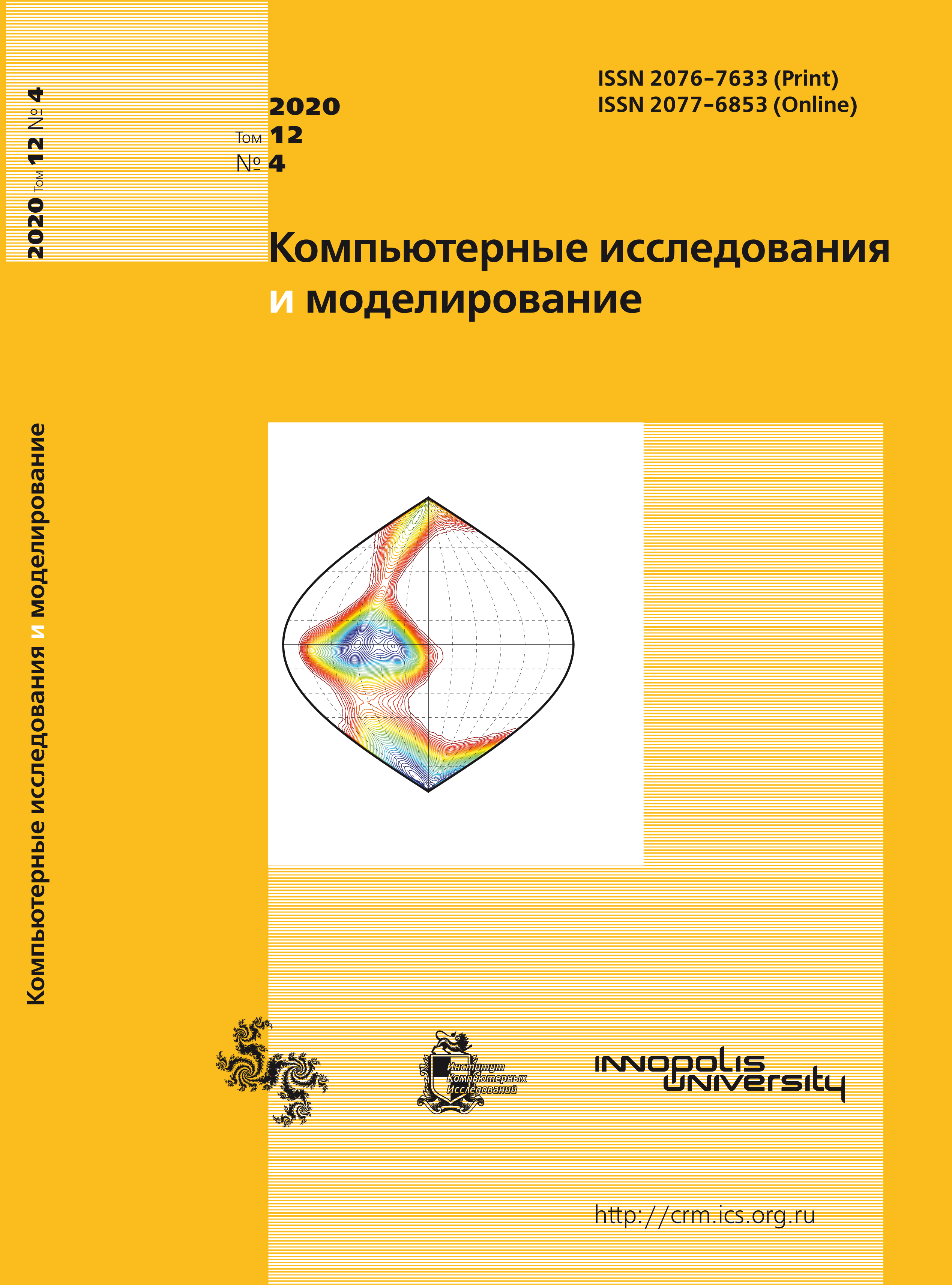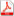Latest issue Issue 4, 2020 Vol. 12

# All issues

Analysis of the basic equation of the physical and statistical approach within reliability theory of technical systemspdf (5122K)

Verification of the physical-statistical approach within reliability theory for the simplest cases was carried out, which showed its validity. An analytical solution of the one-dimensional basic equation of the physicalstatistical approach is presented under the assumption of a stationary degradation rate. From a mathematical point of view this equation is the well-known continuity equation, where the role of density is played by the density distribution function of goods in its characteristics phase space, and the role of fluid velocity is played by intensity (rate) degradation processes. The latter connects the general formalism with the specifics of degradation mechanisms. The cases of coordinate constant, linear and quadratic degradation rates are analyzed using the characteristics method. In the first two cases, the results correspond to physical intuition. At a constant rate of degradation, the shape of the initial distribution is preserved, and the distribution itself moves equably from the zero. At a linear rate of degradation, the distribution either narrows down to a narrow peak (in the singular limit), or expands, with the maximum shifting to the periphery at an exponentially increasing rate. The distribution form is also saved up to the parameters. For the initial normal distribution, the coordinates of the largest value of the distribution maximum for its return motion are obtained analytically.

In the quadratic case, the formal solution demonstrates counterintuitive behavior. It consists in the fact that the solution is uniquely defined only on a part of an infinite half-plane, vanishes along with all derivatives on the boundary, and is ambiguous when crossing the boundary. If you continue it to another area in accordance with the analytical solution, it has a two-humped appearance, retains the amount of substance and, which is devoid of physical meaning, periodically over time. If you continue it with zero, then the conservativeness property is violated. The anomaly of the quadratic case is explained, though not strictly, by the analogy of the motion of a material point with an acceleration proportional to the square of velocity. Here we are dealing with a mathematical curiosity. Numerical calculations are given for all cases. Additionally, the entropy of the probability distribution and the reliability function are calculated, and their correlation is traced.

Keywords: degradation, reliability, nanosystem, physical and statistical approach, entropy, continuity equation
Citation in English: Kozhevnikov V.S., Matyushkin I.V., Chernyaev N.V. Analysis of the basic equation of the physical and statistical approach within reliability theory of technical systems // Computer Research and Modeling, 2020, vol. 12, no. 4, pp. 721-735
Citation in English: Kozhevnikov V.S., Matyushkin I.V., Chernyaev N.V. Analysis of the basic equation of the physical and statistical approach within reliability theory of technical systems // Computer Research and Modeling, 2020, vol. 12, no. 4, pp. 721-735
DOI: 10.20537/2076-7633-2020-12-4-721-735

Full-text version of the journal is also available on the web site of the scientific electronic library eLIBRARY.RU

The journal is included in the Russian Science Citation Index

The journal is included in the List of Russian peer-reviewed journals publishing the main research results of PhD and doctoral dissertations.

International Interdisciplinary Conference "Mathematics. Computing. Education"

The journal is included in the RSCI

Indexed in Scopus